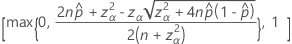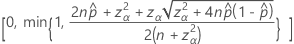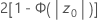# 单比率 的方法和公式

## 精确方法的置信区间 (CI)

### 下限

#### 表示法

v1 2x
v2 2(nx + 1)
x 事件数
n 试验数
F 自由度为 v1v2 的 F 分布的 α/2 下限点

### 上限

#### 表示法

v1 2(x + 1)
v2 2(nx)
x 事件数
n 试验数
F 自由度为 v1v2 的 F 分布的 α/2 上限点

## 正态近似的置信区间 (CI)

### 表示法观测到的概率= x / n
x n 个试验中观测到的事件数
n 试验数
zα/2 标准正态分布在 1–α/2 处的逆累积概率
α 1 – 置信水平/100

## 精确检验

### 公式

• H1p > po，p 值 = P{ X > x | p = po}
• H1p < po，p 值 = P{ X < x | p = po}
• H1ppopo = 1/2，p 值 = P{ X < y or X > ny | p = po}

n 试验数
p 成功的概率
x 观测到的成功次数
y min {x, n x}

## 似然比检验

### 公式

Minitab 将似然比检验用于：

H1：p ≠ po 且 po ≠ 1/2

LR (x) ≥ c

Minitab 求 X = (0, 1,…, n) 的所有可能值的似然比，并求满足 LR (y) ≥ LR (x) 的所有值的概率之和。
• p 值 = Σ P{X = y | p = po}

### 表示法

c 所选择的用于获取所需置信水平 α 的临界值
x 观测到的成功次数
y min {x, nx}
n 试验数

## 正态近似的检验统计量

### 表示法观测到的概率 x/n
x n 个试验中观测到的事件数
n 试验数
p0 假设概率